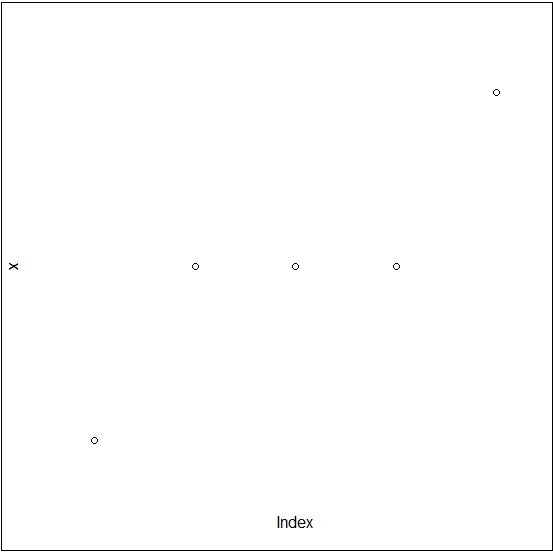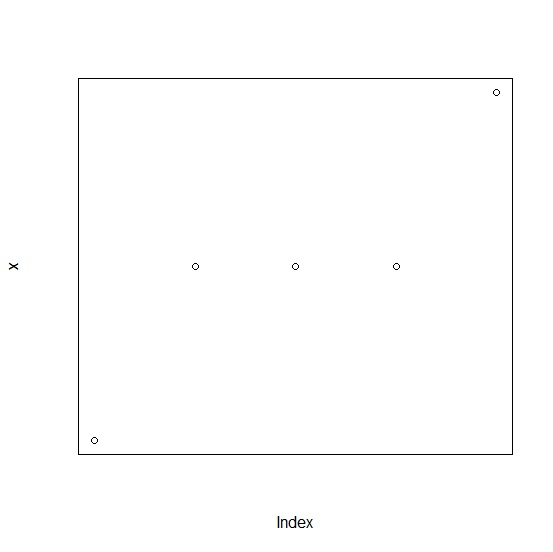# How to create a base R plot without axes but keeping the frame of the plot?

To create a base R plot without axes but keeping the frame of the plot, we can set axes argument to FALSE and frame.plot argument to TRUE.

For example, if we have a vector called V and we want to create a plot of V without axes but with the frame of the plot then, we can use the command given below −

plot(V,axes=FALSE,frame.plot=TRUE)

Check out the below example to understand how it works.

## Example

Consider the following snippet −

x<-rpois(5,2)
plot(x,axes=FALSE)

## Output

If you execute the above given snippet, it generates the following Output −To create a base R plot without axes but keeping the frame of the plot, add the following code to the above snippet −

x<-rpois(5,2)
plot(x,axes=FALSE,frame.plot=TRUE)

## Output

If you execute all the above given snippets as a single program, it generates the following Output −